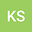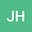loading page

Utilization of the fractal dimension metric in training of dense Neural Networks
••Jordan Hristov
University of Chemical technology and Metallurgy Sofia 1756, 8 Kilment 0hridsky, blud, BULGARIA
Author Profile## Abstract

The process of training of Artificial Neural Networks essentially is optimization of the values of the weights $w_{pq}$ associated with the arcs, connecting the nodes of the layers. This is a process of minimization of the Loss function (maximization of Accuracy function). During the training, the training data set recursively is utilized at subsequent stages, called \textit{Epochs}. The training continues until a satisfactory values of the Loss, Accuracy etc. parameters are reached. The matrices $W^{UV}$ comprising the weights of the arcs connecting the layers $U$ and $V$, can be regarded as gray-scale images of a surface. Starting as random matrices, processed by recursive procedures, they gradually become fractal structures, characterized with respective fractal dimension $D_f$. In the presented article we have made an attempt to utilize the correspondence of $D_f$ with the Loss/Accuracy values, in order to forecast the optimal ending point of the NN training process. Similar conclusions were made for the correspondence between the number of layer’s nodes and $D_f$. An attempt to apply statistically more rigorous approach in the determination of the slope of the regression line in Richardson-Mandelbrot plot, was made.

#### Peer review status:UNDER REVIEW

02 Jun 2021Submitted to Mathematical Methods in the Applied Sciences
03 Jun 2021Assigned to Editor
03 Jun 2021Submission Checks Completed
04 Jun 2021Reviewer(s) Assigned